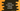# Python numpy interp method example## Python numpy interp method example to calculate one-dimensional piecewise linear interpolant:

In Python, we can use interp() method defined in NumPy to get one-dimensional linear interpolation to a function with given discrete data points.

In this post, I will show you how to use interp() with an example and its definition.

### Definition of interp:

numpy.interp is defined as like below:

``numpy.interp(x, xp, fp, left=None, right=None, period=None)``

Here,

• x is an array_like x-coordinates to evaluate the interpolated values.
• xp are the x-coordinates of the data points and fp are the y-coordinates of the data points. The size of both should be equal.
• left is the value to return for x < xp, and right is the value to return for x > xp[-1]. Both are optional values and by default, these are fp and fp[-1]
• period is the period for the x-coordinates. If it is given, left and right are ignored. This is also optional.

#### Return value of interp:

interp returns the interpolated values.

#### ValueError:

It can raise ValueError if period is 0, if xp or fp has different length or if xp and fp are not one dimensional sequence.

## Example of numpy interp:

Let’s take a look at the below example of numpy.interp:

``````import numpy as np

x = 1.2
xp = [5, 10, 15]
fp = [3, 9, 19]

i = np.interp(x, xp, fp)

print(i)``````

It will 3.0.

Let’s change x to a 1-D array:

``````import numpy as np

x = [1, 2, 4, 6, 8, 9]
xp = [0, 5, 10]
fp = [3, 9, 19]

i = np.interp(x, xp, fp)

print(i)``````

It will print:

``[ 4.2   5.4  7.8 11.  15.  17. ]``

Let me plot the points for the above example to give you a better understanding:

``````import numpy as np
import matplotlib.pyplot as plt

x = [1, 2, 4, 6, 8, 9]
xp = [0, 5, 10]
fp = [3, 9, 19]

i = np.interp(x, xp, fp)

plt.plot(xp, fp, 'o')
plt.plot(x, i, 'o', alpha=0.5)

plt.show()``````